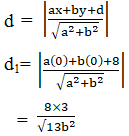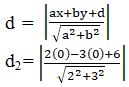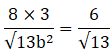For what values of a and b the intercepts cut off on the coordinate axes by the line ax + by + 8 = 0 are equal in length but opposite in signs to those cut off by the line 2x – 3y + 6 = 0 on the axes.

Asked by Aaryan | 1 year ago |  26

##### Solution :-

Given:

Intercepts cut off on the coordinate axes by the line ax + by +8 = 0 …… (i)

And are equal in length but opposite in sign to those cut off by the line

2x – 3y +6 = 0 ……(ii)

We know that, the slope of two lines is equal

The slope of the line (i) is $$\dfrac{-a}{b}$$

The slope of the line (ii) is $$\dfrac{2}{3}$$

So let us equate,

$$\dfrac{-a}{b}= \dfrac{2}{3}$$

a = $$\dfrac{-2b}{3}$$

The length of the perpendicular from the origin to the line (i) is

By using the formula,The length of the perpendicular from the origin to the line (ii) is

By using the formula,It is given that, d1 = d2b = 4

So, a = $$\dfrac{-2b}{3}$$

$$\dfrac{-8}{3}$$

The value of a is $$\dfrac{-8}{3}$$ and b is 4.

Answered by Aaryan | 1 year ago

### Related Questions

#### Find the angle between the line joining the points (2, 0), (0, 3) and the line x + y = 1.

Find the angle between the line joining the points (2, 0), (0, 3) and the line x + y = 1.

#### Prove that the points (2, -1), (0, 2), (2, 3) and (4, 0) are the coordinates of the vertices

Prove that the points (2, -1), (0, 2), (2, 3) and (4, 0) are the coordinates of the vertices of a parallelogram and find the angle between its diagonals.

#### Find the acute angle between the lines 2x – y + 3 = 0 and x + y + 2 = 0.

Find the acute angle between the lines 2x – y + 3 = 0 and x + y + 2 = 0.